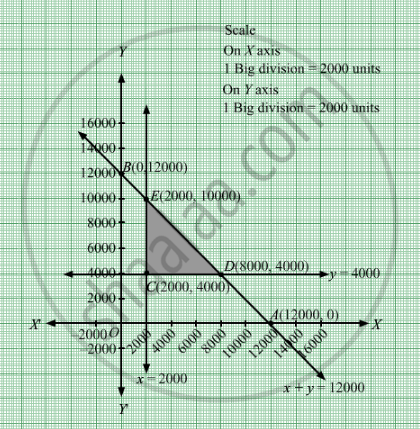# Anil Wants to Invest at Most Rs 12000 in Saving Certificates and National Saving Bonds. , He Has to Invest at Least Rs 2000 in Saving Certificates and at Least Rs 4000 in National Saving Bonds - Mathematics

Sum

Anil wants to invest at most Rs 12000 in Saving Certificates and National Saving Bonds. According to rules, he has to invest at least Rs 2000 in Saving Certificates and at least Rs 4000 in National Saving Bonds. If the rate of interest on saving certificate is 8% per annum and the rate of interest on National Saving Bond is 10% per annum, how much money should he invest to earn maximum yearly income? Find also his maximum yearly income.

#### Solution

Let Anil invests Rs x  in Saving certificates and Rs y in National Saving bonds.
Therefore ,

$x, y \geq 0$

Anil wants to invest at most Rs 12000 in Saving Certificates and National Saving Bonds.

$x + y \leq 12000$

According to rules, he has to invest at least Rs 2000 in Saving Certificates and at least Rs 4000 in National Saving Bonds.

$x \geq 2000$

$y \geq 4000$

If the rate of interest on saving certificate is 8% per annum and the rate of interest on National Saving Bond is 10% per annum.

Total earning from investment = Z = $\frac{8x}{100} + \frac{10y}{100}$  which is to be maximised.

Thus, the mathematical formulat​ion of the given linear programmimg problem is

Max Z =$\frac{8x}{100} + \frac{10y}{100}$

subject to

$x + y \leq 12000$
$x \geq 2000$
$y \geq 4000$

$x, y \geq 0$

First we will convert inequations into equations as follows:
+ y =12000, x = 2000, = 4000, x = 0 and y = 0

Region represented by x y ≤ 12000:
The line x + y = 12000 meets the coordinate axes at A(12000, 0) and B(0, 12000) respectively. By joining these points we obtain the line x + y = 12000. Clearly (0,0) satisfies the inequation x y ≤ 12000. So,the region which contains the origin represents the solution set of the inequation x y ≤ 12000.

Region represented by x  ≥ 2000:
The line x  = 2000 is the line that passes through (2000, 0) and is parallel to Y axis.The region to the right of the line x  = 2000 will satisfy the inequation x  ≥ 2000.

Region represented by  ≥ 4000:
The line y = 4000 is the line that passes through (0, 4000) and is parallel to X axis.The region above the line y = 4000 will satisfy the inequation ≥ 4000.

Region represented by ≥ 0 and y ≥ 0:
Since, every point in the first quadrant satisfies these inequations. So, the first quadrant is the region represented by the inequations x ≥ 0, and y ≥ 0.

The feasible region determined by the system of constraints isThe corner points are E(2000, 10000), D(8000, 4000), C(2000, 4000)

The values of Z at these corner points are as follows

 Corner point Z=$\frac{8x}{100} + \frac{10y}{100}$ E 1160 D 1040 C 560

The maximum value of Z is 1160 which is attained at E(2000, 10000.
Thus, the maximum earning is Rs 1160 obtained when Rs 2000 were invested in Saving's certificates and Rs 10000 were invested in National Saving Bond.

Concept: Graphical Method of Solving Linear Programming Problems
Is there an error in this question or solution?

#### APPEARS IN

RD Sharma Class 12 Maths
Chapter 30 Linear programming
Exercise 30.4 | Q 24 | Page 53